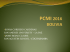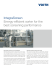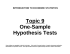# One-Sample Hypothesis Tests Review Problems

## Transcription

One-Sample Hypothesis Tests Review Problems
```One-Sample Hypothesis Tests Review Problems
1.
2.
3.
4.
5.
6.
7.
8.
9.
10.
11.
12.
13.
14.
15.
16.
At the 0.05 significance level, test the claim that the proportion of defects p for a certain product equals 0.3. Sample
data consist of n = 100 randomly selected products, of which 45 are defective.
At the 0.01 level of significance, test the claim that the proportion of voters p who favor nuclear disarmament is less
than 0.7. Sample data consist of 50 randomly selected voters, of whom 31 favor nuclear disarmament.
A manufacturer considers his production process to be out of control when defects exceed 3%. A random sample of
500 items includes exactly 22 defects, but the manager claims that this represents a chance fluctuation and that
production is not really out of control. Test the manager's claim at the 5% level of significance.
The mathematics department has had a consistent failure rate of 12%. In one experimental semester, more active
counseling plus rigorous enforcement of prerequisites results in 338 failures among 3217 mathematics students. At
the 0.05 significance level, test the department's claim that the failure rate has been lowered.
In a genetics experiment, the Mendelian law is followed as expected if one-eighth of the offspring exhibit a certain
recessive trait. Analysis of 500 randomly selected offspring indicates that 83 exhibited the necessary recessive trait.
Is the Mendelian law being followed as expected? Use a 2% level of significance.
At the 0.01 level of significance, test the claim that more than 1/3 of all adults smoke. A random sample of 324 adults
shows that 120 smoke.
In a poll of 1553 randomly selected adult Americans, 92% recognize the name Christopher Columbus. At the 0.01
significance level, test the claim that 95% of all adult Americans recognize the name Columbus.
The unemployment rate was 8.2% in February. In March the Bureau of the Census polled 50,000 randomly selected
employable subjects. Of these, 3785 subjects were classified as unemployed. At the 5% level of significance, test
the assertion of the labor leader who claimed that the March unemployment rate was not below the February rate.
In a study of 500 aircraft accidents involving spatial disorientation of the pilot, it was found that 91% of those
accidents resulted in fatalities. At the 0.05 level of significance, test the claim that three-fourths of all such accidents
will result in fatalities.
Test the claim that fewer than 1/2 of San Francisco residential telephones have unlisted numbers. A random sample
of 400 such phones results in an unlisted rate of 39%. Use a 0.01 level of significance.
The null hypothesis should include the condition of equality, while the alternative hypothesis should involve one of
the three signs >, <, or ≠. For each of the following claims, identify H0 and Ha:
a.
age of professors is more than 30 years.
b.
I.Q. of criminals is above 100.
c.
I.Q. of college students is at least 100.
d.
annual household income is at least \$12,300.
e.
monthly maintenance cost of an aircraft is \$3,271.
f.
height of females is 1.6 meters.
g.
annual salary of air traffic controllers is more than \$26,000
h.
The mean annual salary of college presidents is under \$50,000,
i.
The mean weight of girls at birth is at most 3.2 kilograms.
j.
The mean life of a car battery is not more than 46 months.
Identify the type I error and the type II error corresponding to each claim in Exercise 11.
Weatherwise is a magazine published in association with the American Meteorological Society. In the January 1994
issue there is a rating system to classify Nor'easter storms that frequently hit New England states and can cause
much damage near the ocean coasts. A seven storm has an average peak wave height of 16.4 feet for waves hitting
the shore. Suppose that a Nor'easter is in progress at the severe storm class rating. Peak wave heights are usually
measured from land (using binoculars) off fixed cement peers. Suppose that a reading of 36 peak waves showed an
average wave height of =15.1 feet with sample standard deviation s = 3.2 feet. Does this information indicate that
the storm is (perhaps temporarily) retreating from its severe rating? Use α = 0.01.
USA Today (July 16, 1993) reported that automobile plants in the United States required an average of 24.9 hours to
assemble a new car. In order to reduce inventory costs, a new "just-in-time” parts availability has been introduced on
the assembly line. Suppose that a random sample of 49 cars showed a sample mean assembly time under the new
system was
= 25.2 hours with sample standard deviation s = 1.6 hours. Does this information indicate that the
population mean assembly time is different (either higher or lower) under the new system? Use α = 0.05.
Maureen is a cocktail hostess in a very exclusive private club. The Internal Revenue Service is auditing her tax
return this year. Maureen claims that her average tip last year was \$4.75. To support this claim, she sent the IRS a
random sample of 52 credit card receipts showing her bar tips. When the IRS got the receipts, they computed the
sample average and found it to be
= \$5.25 with sample standard deviation s = \$1.15. Do these receipts indicate
that the average tip Maureen received last year was more than \$4.75? Use a 1% level of significance.
Consumer Reports (March 1993) indicated that the mean acceleration time (0 to 60 miles per hour) for the Dodge
Intrepid was 10.2 seconds. In most tests of this type, regular unleaded gasoline is used. Suppose that 41 such tests
were made using premium unleaded gasoline (octane over 91), and the sample mean acceleration time (0 to 60
17.
18.
19.
20.
21.
miles per hour) was
= 9.7 seconds with sample standard deviation s = 2.1 seconds. Does this indicate that
premium gasoline tends to reduce average acceleration time (0 to 60 miles per hour)? Use α = 0.05.
The Statistical Abstract of the United States (109th edition) reported that the average cost per day of owning an
automobile in the United States is \$7.62. This includes the cost of the car, general maintenance, gasoline, and
insurance. A random sample of 54 college students who own cars found the average cost per day to be \$6.78 with
standard deviation \$1.77. Use a 1% level of significance to test the claim that college students' average daily
ownership expenses are less than the national average.
In 1981, the mean age for the deer population (excluding fawns) in Mesa Verde National Park was 3.97 years.
Suppose that this past year a random sample of 76 adult deer showed the sample mean age to be
= 4.23 years
with sample standard deviation s = 1.82 years. Does this information indicate that the population mean age of deer
in Mesa Verde National Park is different from that in 1981 (either higher or lower)? Use α = 0.05.
The 100 Best Mutual Funds You Can Buy is a book by G. K. Williamson. In this book, Williamson points out that you
do not need to be rich to buy shares and profit from a mutual fund. One of the funds described in the book is the
Janus Growth Fund. This mutual fund was begun in 1970 and since then has averaged 1.43% growth per month (or
17.2% per year) up to 1994. Suppose that you buy tome shares in the Janus Growth Fund and for 36 months you
find that the sample mean growth per month is
= 1.51% with sample standard deviation s = 0.38%. Does this indicate that the population mean growth rate is different (either up or down) from the long-term average growth rate?
Use α = 0.05.
An Air Force base mess hall has received a shipment of 10,000 gallon-size cans of cherries. The supplier claims that
the average amount of liquid is 0.25 gallon per can. A government inspector took a random sample of 100 cans and
found the average liquid content to be 0.28 gallon per can with a standard deviation of 0.10. Does this indicate that
the supplier's claim is too low? (Use a 5% level of significance.)
Is there a relationship between confidence intervals and two-tailed hypothesis tests? Let c be the level of confidence
used to construct a confidence interval from sample data. Let α be the level of significance for a two-tailed
hypothesis test. The following statement applies to hypothesis tests of the mean (both large and small samples).
For a two-tailed hypothesis test with level of significance α and null hypothesis H0: µ = k, we reject H0 whenever k
falls outside the c = 1 - α confidence interval for µ based on the sample data. When k falls within the c = 1 - α
confidence interval, we do not reject H0.
Whenever the value of k given in the null hypothesis falls outside the c = 1 - α confidence interval for the parameter,
we reject H0. For example, consider a two-tailed hypothesis test with α = 0.01 and H0: µ = 20 and Ha: µ ≠ 20.
A random sample of size 36 has a sample mean
= 22 with sample standard deviation s = 4.
(a) What is the value of c = 1 - α? Construct a 1 - α confidence interval for p from the sample data. What is the
value of µ given in the null hypothesis (i.e., what is k)? Is this value in the confidence interval? Do we reject or fail to
reject H0 based on this information?
(b) Find the critical region for the hypothesis test. Do we reject or fail to reject H0?
Compare your result to that of part a.
One-Sample Hypothesis Tests Review Problems
1.
Test statistic: z = 3.27. Critical values: z = -1.96 and z = 1.96. Reject H0: p = 0.3.
There is sufficient sample evidence to warrant rejection of the claim that the proportion of defects is equal to 0.3. It
seems to be significantly higher.
2.
Test statistic: z = -1.23. Critical value: z = -2.33. Fail to reject H0: p = 0.7.
There is not sufficient sample evidence to support the claim that the proportion of voters who favor nuclear
disarmament is less than 0.7.
3.
Test statistic: z = 1.84. Critical value: z = 1.645. Reject H0: p = 0.03. Reject the manager's claim that production is
not out of control; it appears that production is out of control.
4.
Test statistic: z = -2.61. Critical value: z = -1.645. Reject H0: p = 0.12. The sample data support the claim that the
failure rate has been lowered from the 12% level.
5.
Test statistic: z = 2.77. Critical values: z = -2.33 and z = 2.33. Reject H0: p = 1/8.
The Mendelian law does not appear to be working since the sample results differ significantly from the 1/8 proportion
that was expected.
6.
Test statistic: z = 1.41. Critical value: z = 2.33. Fail to reject H0: p = 1/3.
There is not sufficient sample evidence to support the claim that more than 1/3 of all adults smoke.
7.
Test statistic: z = -5.40. Critical values: z = -2.575 and z = 2.575. Reject H0: p = 0.95.
There is sufficient sample evidence to warrant rejection of the claim that 95% recognize Columbus. In fact, the rate
appears to be lower than 95%.
8.
Test statistic: z = -5.13. Critical value: z = -1.645. Reject H0: p = 0.082.
Reject the labor leader's claim that the rate was at least 8.2%. The sample data indicate a rate that is significantly
less than 8.2%. (The sample rate is 7.57 %.)
9.
Test statistic: z = 8.26. Critical values: z = -1.96 and z = 1.96. Reject H0: p = 3/4.
The sample rate of 91% differs significantly from the claimed rate of 3/4. Reject the claim that 3/4 of all such
accidents will result in fatalities.
10. Test statistic: z = -4.40. Critical value: z = -2.33. Reject H0: p = 1/2. The sample rate of 39% is significantly less than
the claimed rate of 1/2 or 50%. There is sufficient sample evidence to support the claim that fewer than 1/2 of San
Francisco residential telephones have unlisted numbers.
11. a.
b.
c.
d.
e.
f.
g.
h.
i.
12.
j.
a. Type I = age of professors is more than 30 years, when in fact, age of professors is 30 years.
Type II = age of professors is 30 years, when in fact, age of professors is more than 30 years.
b. Type I = I.Q. of criminals is above 100, when in fact, I.Q. of criminals is 100.
Type II = I.Q. of criminals is 100, when in fact, I.Q. of criminals is above 100
c. Type I = I.Q. of college students is less than 100, when in fact; I.Q. of college students is at least 100.
Type II = I.Q. of college students is at least 100, when in fact; I.Q. of college students is less than 100.
d. Type I = annual household income is less than \$12,300, when in fact; annual household income is at least
\$12,300.
Type II = annual household income is at least \$12,300, when in fact, annual household income is less than
\$12,300
e. Type I = monthly maintenance cost of an aircraft is not \$3,271, when in fact; monthly maintenance cost of an
aircraft is \$3,271.
Type II = monthly maintenance cost of an aircraft is \$3,271, when in fact; monthly maintenance cost of an
aircraft is not \$3,271.
f. Type I = height of females is not 1.6 meters, when in fact, height of females is 1.6 meters.
Type II = height of females is 1.6 meters, when in fact; height of females is not 1.6 meters.
g. Type I = annual salary of air traffic controllers is more than \$26,000, when in fact; annual salary of air traffic
controllers is \$26,000.
Type II = annual salary of air traffic controllers is \$26,000, when in fact; annual salary of air traffic controllers
is more than \$ 26,000.
h. Type I = The mean annual salary of college presidents is under \$50,000, when in fact, the mean annual salary
of college presidents is \$50,000.
Type II = The mean annual salary of college presidents is \$50,000, when in fact, the mean annual salary of
college presidents is under \$50,000
i. Type I = The mean weight of girls at birth is more than 3.2 kilograms, when in fact; the mean weight of girls at
birth is at most 3.2 kilograms.
Type II = The mean weight of girls at birth is at most 3.2 kilograms, when in fact, the mean weight of girls at
birth is more than 3.2 kilograms.
j. Type I = The mean life of a car battery is not more than 46 months, when in fact, the mean life of a car battery is
46 months.
Type II = The mean life of a car battery is 46 months, when in fact, the mean life of a car battery is not more
than 46 months
13.
14.
15.
16.
17.
18.
19.
20.
21.
H0: µ = 16.4; Ha: µ < 16.4; left-tailed test; t = -2.4377; t value corresponding to
is t = -2.4375. Since the sample
test statistic falls to the right of the critical value in the critical region, we fail to reject H0. The storm seems to be not
retreating from its severe rating.
H0: µ = 24.9; Ha: µ ≠ 24.9; two-tailed test; ±t = ±2.01; t value corresponding to
is t = 1.31. Since the sample test
statistic does not fall in the critical region, we fail to reject H0. There is not enough evidence to conclude that the
assembly time under the new system is different from that under the old system.
H0: µ = 4.75; Ha: µ > 4.75; right-tailed test; t = 2.40; t value corresponding to
is t = 3.14. Since the sample test
statistic falls in the critical region, we reject H0. It appears that the average tip Maureen received was greater than
\$4.75.
H0: µ = 10.2; Ha: µ < 10.2; left-tailed test; t = -1.68; t value corresponding to
is t = -1.52. Since the sample test
statistic does not fall in the critical region, we fail to reject H0. There is not enough evidence to conclude that the
average acceleration time for cars using premium gasoline is less than that for cars using regular.
H0: µ = 7.62; Ha: µ < 7.62; left-tailed test; t = -2.40; t value corresponding to
is t = -3.49. Since the sample test
statistic falls in the critical region, we reject H0. The average daily cost of owning a car for a college student seems
to be less than the reported national average cost.
H0: µ = 3.97; Ha: µ ≠ 3.97; two-tailed test; ±t = ±1.99; t value corresponding to
is t = 1.25. Since the sample test
statistic does not fall in the critical region, we fail to reject H0. We do not have enough evidence to conclude that the
mean age of deer in Mesa Verde National Park has changed since 1981.
H0: µ =1.43; Ha: µ ≠ 1.43; two-tailed test; ±t = ±2.03; t value corresponding to
is t = 1.26. Since the sample test
statistic does not fall in the critical region, we fail to reject H0. There is not enough evidence to conclude that the
population mean growth rate is different from the long-term average growth rate.
H0: µ = 0.25; Ha: µ > 0.25; right-tailed test; t = 1.66; t value corresponding to
is t = 3.0. Since the sample test
statistic falls in the critical region, we reject H0. The supplier's claim that the average amount of liquid is 0.25 gallon
per can seems to be too low.
(a) c = 0.99; a 99% confidence interval for µ is from 20.18 to 23.82; H0 states µ = 20, and 20 is not in the
confidence interval, so we reject H0.
(b) ±t = ±2.72; t value corresponding to
is t = 3.00. Since the sample test statistic falls in the critical region, we
reject H0. This is the same conclusion we made in part a.
```

### HYPOTHESIS TESTING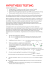### Document 6537334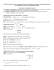### File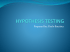### Job Search Form - Service Canada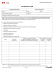### Stat 100 Sample Final Questions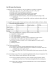### chapter 9 practice - faculty.piercecollege.edu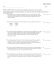### What is Hypothesis Testing? AP Statistics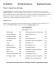### Inferential Statistics t and ScWk 242 – Session 9 Slides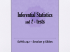### STAT 2000 Sample Midterm (actually given in October 2007)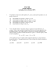### How to write a Research Grant? or Spencer Gibson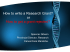### Elementary Statistics Sample Exam #3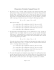### Sampling Distributions and One Sample Tests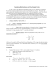### 3.3 Statistical Inference with one sample from a population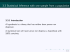### sonia cordero cardenas san andres university – la paz •jaime rojas